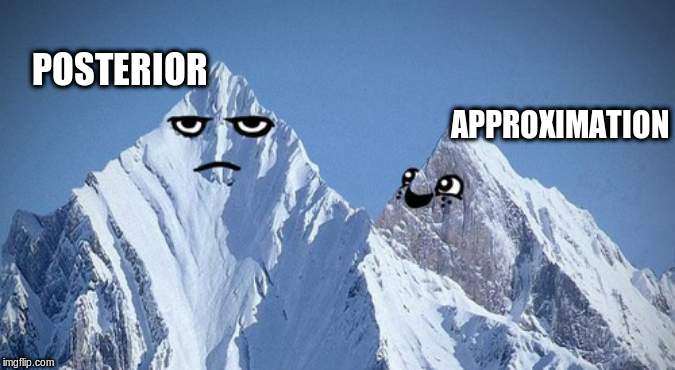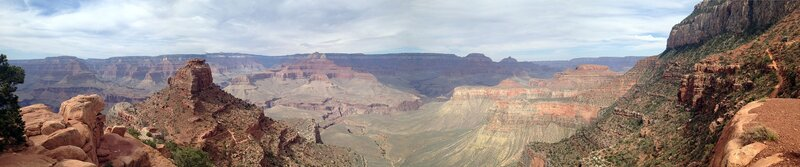# Another normalizing flow: Inverse Autoregressive Flows

## November 12, 2019This post we will explore a type of normalizing flow called Inverse Autoregressive Flow. A composition (flow) of transformations, while preserving the constraints of a probability distribution (normalizing), can help us obtain highly correlated variational distributions. Don’t repeat yourself If what was mentioned in the previous lines didn’t ring a bell, do first read these posts: variational inference and normalizing flows. This post could really be seen as an extension of the latter.

# Distribution estimation with Masked Autoencoders

## October 25, 2019Four of my last five blog posts were more or less related to Baysian inference with variational methods. I had some momentum, and I wanted to use the traction I gained to do another post (which will come!) on enhancing variational methods with Inverse Autoregressive Flows (IAF), but first I have to get something different out of the way. In the paper describing IAF, they refer to an autoregressive neural network (and further assume his to be clear knowlegde).

# Sculpting distributions with Normalizing Flows

## October 11, 2019Last posts we’ve investigated Bayesian inference through variational inference (post 1/post 2). In Bayesian inference, we often define models with some unknown model parameters $Z$, or latent stochastic variables $Z$. Given this model and some observed data points $D = \{ D_1, D_2, \dots, D_n \}$, we are interested in the true posterior distribution $P(Z|D)$. This posterior is often intractable and the general idea was to forgo the quest of obtaining the true posterior, but to accept that we are bounded to some easily parameterizable approximate posteriors $^*Q(z)$, which we called variational distributions.

# Variational inference from scratch

## September 16, 2019In the posts Expectation Maximization and Bayesian inference; How we are able to chase the Posterior, we laid the mathematical foundation of variational inference. This post we will continue on that foundation and implement variational inference in Pytorch. If you are not familiar with the basis, I’d recommend reading these posts to get you up to speed. This post we’ll model a probablistic layer as output layer of a neural network.

# Algorithm Breakdown: Bayesian Optimization

## August 25, 2019Not that long ago I wrote an introduction post on Gaussian Processes (GP’s), a regression technique where we condition a Gaussian prior distribution over functions on observed data. GP’s can model any function that is possible within a given prior distribution. And we don’t get a function $f$, we get a whole posterior distribution of functions $P(f|X)$. This of course, sounds very cool and all, but there is no free lunch.

# Bayesian inference; How we are able to chase the Posterior

## June 10, 2019Bayesian modeling! Every introduction on that topic starts with a quick conclusion that finding the posterior distribution often is computationally intractable. Last post I looked at Expectation Maximization, which is a solution of this computational intractability for a set of models. However, for most models, it isn’t. This post I will take a formal definition of the problem (As I’ve skipped that in the Expectation Maximization post) and we’ll look at two solutions that help us tackle this problem; Markov Chain Monte Carlo and Variational Inference.

# Algorithm Breakdown: Expectation Maximization

## May 24, 2019I wanted to learn something about variational inference, a technique used to approximate the posterior distribution in Bayesian modeling. However, during my research, I bounced on quite some mathematics that led me to another optimization technique called Expectation Maximization. I believe the theory behind this algorithm is a stepping stone to the mathematics behind variational inference. So we tackle the problems one problem at a time!

# Fully automated soil classification with a Convolutional Neural Network and Location embeddings

## April 2, 2019Soil classification is, in practice, a human process. A geotechnical engineer interprets results from a Cone Penetration Test and comes up with a plausible depiction of the existing soil layers. These interpretations will often be used throughout a project and are input for many following calculations. Just as the poliovirus, the process of manually mapping data from $x$ to $y$, belongs to the list of things that humanity tries to eradicate from earth.

# Save some time: Embedding jupyter notebook in an iframe and serve as a reverse proxy behind NGINX

## March 17, 2019Embedding Jupyter notebook/ lab on your website can be done by embedding it in an iframe. However, it takes some configurational quirks to get it done. For my purpose, I also needed to offload validation to another service on the backend. Both the validation server as the jupyter notebook server were proxied behind an NGINX server. Here is the configuration. NGINX setup In the configuration, we set two upstream servers.

# An intuitive introduction to Gaussian processes

## February 1, 2019Christopher Fonnesbeck did a talk about Bayesian Non-parametric Models for Data Science using PyMC3 on PyCon 2018. In this talk, he glanced over Bayes’ modeling, the neat properties of Gaussian distributions and then quickly turned to the application of Gaussian Processes, a distribution over infinite functions. Wait, but what?! How does a Gaussian represent a function? I did not understand how, but the promise of what these Gaussian Processes representing a distribution over nonlinear and nonparametric functions really intrigued me and therefore turned into a new subject for a post.

(c) 2019 Ritchie Vink.